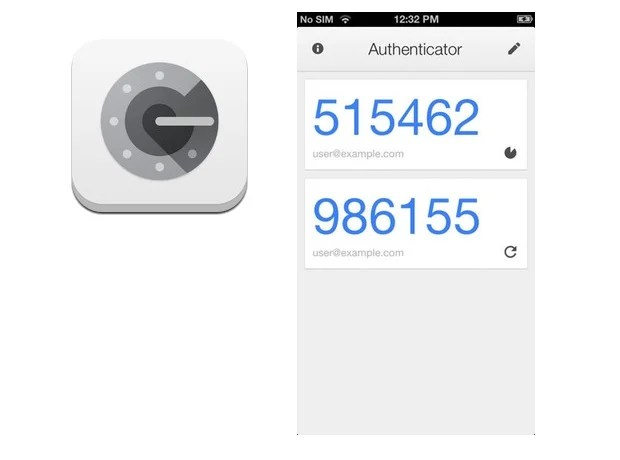Most people use Google Authenticator to generate two-factor authentication (2FA) tokens on their phone, with Authy as a recent alternative. What’s cool is that any service can make use of these apps as long as it is compatible. But what does it mean to be compatible? How do these apps work?Apps like Google Authenticator implement the Time-Based One-Time Password (TOTP) algorithm. It has the following ingredients:

• A shared secret (a sequence of bytes)
• An input derived from the current time
• A signing function

## Shared Secret

The shared secret is what you need to obtain to set up the account on your phone. Either you take a photo of a QR code using your phone or you can enter the secret manually. Because not all byte values are displayable characters the secret is base32-encoded (why not base64?).

For manual entry Google’s services present this secret has the following format:

``````xxxx xxxx xxxx xxxx xxxx xxxx xxxx xxxx
``````

This value is 256 bits but can be smaller for other services. The QR code contains this same token as a URL:

``````otpauth://totp/Google%3Ayourname@gmail.com?secret=xxxx&issuer=Google
``````

## Input (Current Time)

The input time value you’ll simply get from your phone, no further interaction with the server is required once you have obtained the secret. However it is important that your phone’s time is accurate as the server will essentially repeat what happens on your phone using the current time as known by the server.

More specifically the server will actually compare submitted tokens to all tokens generated for a window of time (e.g. a couple of minutes) to account for the time it takes for you to type the token and send it to the server.

## Signing Function

The signing function used is HMAC-SHA1. HMAC stands for Hash-based message authentication code and it is an algorithm that uses a secure one-way hash function (SHA1 in this case) to sign a value. Using an HMAC allows us to verify authenticity – only people knowing the secret can generate the same output for the same input (the current time). This all sounds complex but the algorithm is very simple (details omitted):

``````hmac = SHA1(secret + SHA1(secret + input))
``````

As an aside TOTP is in fact a superset of HOTP or HMAC-Based One-Time Password Algorithm – they are the same thing except that TOTP specifies that the current time is used as the input value while HOTP simply uses an incrementing counter that needs to be synchronized.

## Algorithm

First we’ll need to base32 decode the secret. Google presents it with spaces and in lowercase to make it easier to grok for humans, but base32 actually does not allow spaces and only allows uppercase letters. Thus:

``````original_secret = xxxx xxxx xxxx xxxx xxxx xxxx xxxx xxxx
secret = BASE32_DECODE(TO_UPPERCASE(REMOVE_SPACES(original_secret)))
``````

Next we derive the input from the current time, for this we’ll use UNIX time, or the amount of seconds since the epoch:

``````input = CURRENT_UNIX_TIME()
``````

One thing you have probably noticed in Google Authenticator is that codes are valid for some time before changing to the next value. If the value would change every second it would be a bit difficult to copy, after all. This value defaults to 30 seconds, we can simply do an integer divide by 30 to get a value that will remain stable in a 30 second time window. We don’t really care if the value has a particular scale, as long as the value is reproducible on both sides.

``````input = CURRENT_UNIX_TIME() / 30
``````

Finally we apply the signing function, HMAC-SHA1:

``````original_secret = xxxx xxxx xxxx xxxx xxxx xxxx xxxx xxxx
secret = BASE32_DECODE(TO_UPPERCASE(REMOVE_SPACES(original_secret)))
input = CURRENT_UNIX_TIME() / 30
hmac = SHA1(secret + SHA1(secret + input))
``````

Now, we could be done here as what we have so far will provide effective 2FA. However the resulting HMAC value is a standard-length SHA1 value (20 bytes, 40 hex characters) and nobody wants to type 40 characters. We want to those pretty 6-digit numbers!

To convert the 20-byte SHA1 to a 6-digit number we’ll first slim it down a bit. We will use the last 4 bits of the SHA1 (a value ranging from 0-15) to index into the 20-byte value and use the next 4 bytes at that index. The maximum potential value of this indexing operation is 15 + 4 = 19, which is also the maximum index possible (remember, zero-based) so that will always work. So anyway, we get those 4 bytes:

``````four_bytes = hmac[LAST_BYTE(hmac):LAST_BYTE(hmac) + 4]
``````

We can now turn these into a standard 32 bit unsigned integer (4 bytes = 32 bit).

``````large_integer = INT(four_bytes)
``````

Now we have a number, much better! However as the name suggests, this could still be a very large value (2^32 – 1), and that would obviously not be a 6 digit number. We can guarantee a 6-digit number by using the remainder of dividing by the first 7 digit number. Which is one million.

``````large_integer = INT(four_bytes)
small_integer = large_integer % 1,000,000
``````

This is our final value. Here’s everything together:

``````original_secret = xxxx xxxx xxxx xxxx xxxx xxxx xxxx xxxx
secret = BASE32_DECODE(TO_UPPERCASE(REMOVE_SPACES(original_secret)))
input = CURRENT_UNIX_TIME() / 30
hmac = SHA1(secret + SHA1(secret + input))
four_bytes = hmac[LAST_BYTE(hmac):LAST_BYTE(hmac) + 4]
large_integer = INT(four_bytes)
small_integer = large_integer % 1,000,000
``````